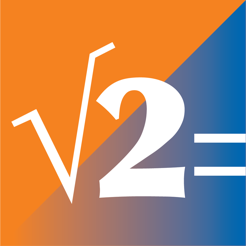## Снимки экрана

•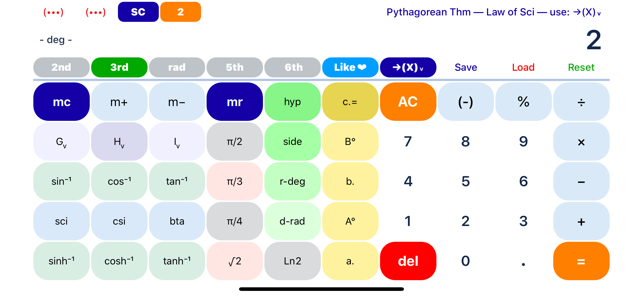•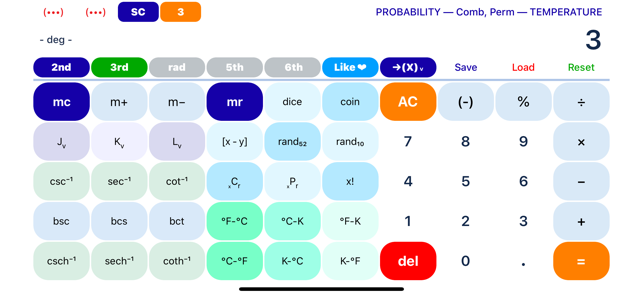•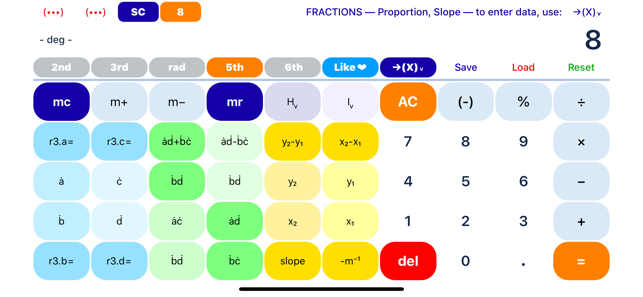•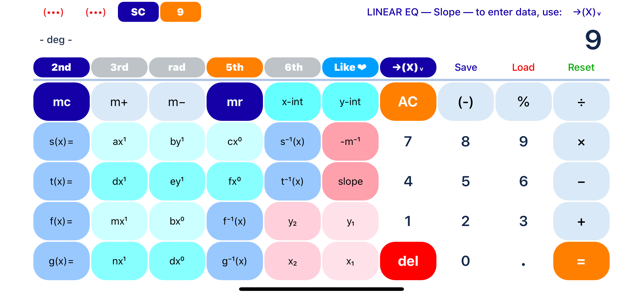•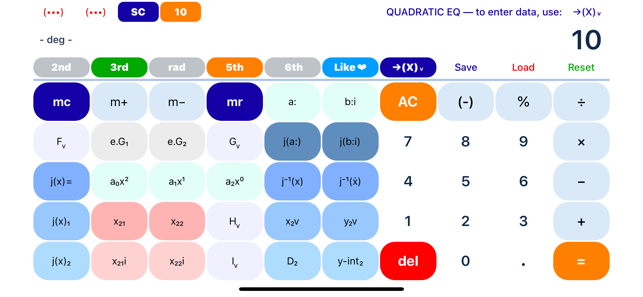•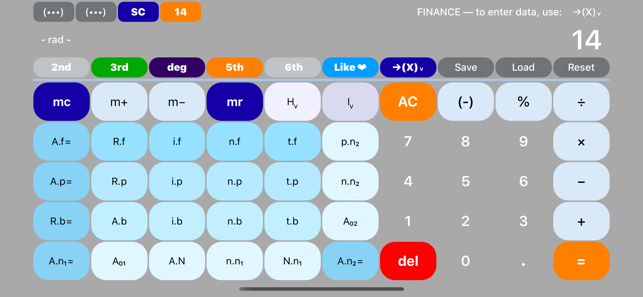•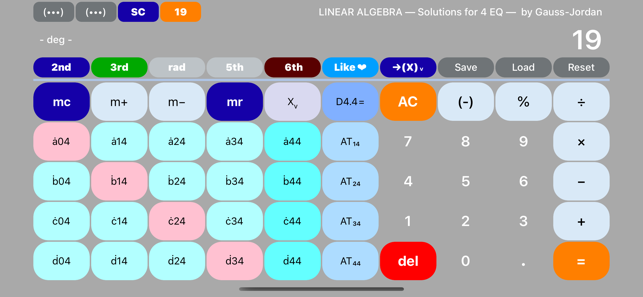•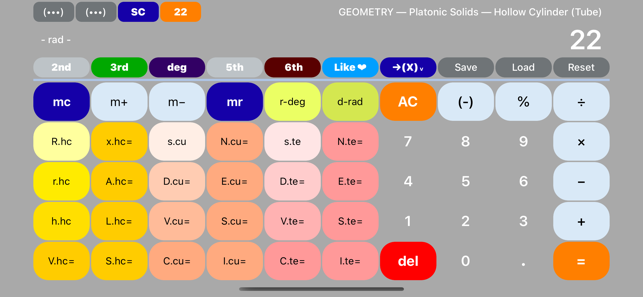•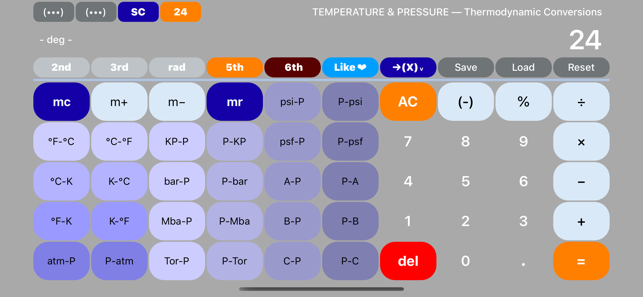•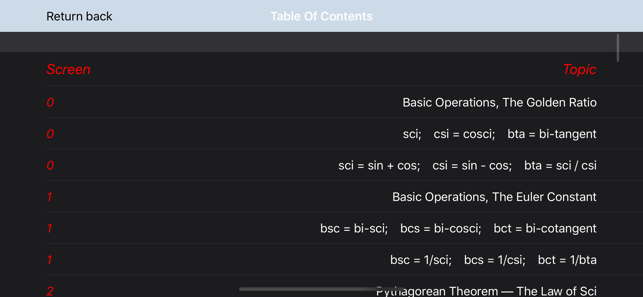## Описание

"SciPro Math" is an extraordinary, polymorphic, scientific calculator, designed for portable devices, that contains hundreds of mathematical operations and has a great capability to store data. It can be used for doing unit conversions, algebra, geometry, trigonometry, pre-calculus, linear algebra, probability, and finance. It has been designed for both students and professionals.

FOR TUTORIALS, GO TO:

BENEFITS:
Introducing SciPro Math, the first Polymorphic Calculator. With the help of function keys to navigate, users discover that the App contains 16 different screens filled with all kinds of exotic mathematical operations. It works like magic.

Using storage variables to hold and store the values of the coefficients, you are now able to enter entire linear, quadratic, cubic, and even quartic equations right on the application itself, and save, solve, and evaluate them, live.

There is no need to write that much. Just solve and store the solutions right on the calculator. It has a great storage capability available for you to use.

Use the calculator for doing unit conversions within a particular system of measurements, but also for performing unit conversions across different systems, namely: the imperial and metric.

Instead of doing complex calculations by hand, like evaluating equations, avoid making silly mistakes, let "SciPro Math" do them for you.

TOPICS:
"SciPro Math" is the perfect companion for your mathematics class. Consider it for the following topics and subjects:
• Arithmetic
• Conversions
• Algebra
• Linear Algebra
• Geometry
•  Trigonometry
•  Probability
• Precalculus
• Finance

FEATURES:
•  Screen identification numbers: 0 – 24
•  A new navigation system that uses the screen numbers: 0 – 24
•  Screen numbers, titles, descriptions, and functional instructions, as follows:
•  0 – Basic Operations — to store data, use: ->(X)v
•  1 – Basic Operations — to store data, use: ->(X)v
•  2 – Pythagorean Thm — Law of Sci — to enter, use: ->(X)v
•  3 – PROBABILITY — Comb, Perm — TEMPERATURE
•  4 – Mixed Conversion — to store data, use: ->(X)v
•  5 – Measure Conversion — to store data, use: ->(X)v
•  6 – Volume Conversion — to store data, use: ->(X)v
•  7 – Weight Conversion — to store data, use: ->(X)v
•  8 – FRACTIONS — Proportion, Slope — to enter data, use: ->(X)v
•  9 – LINEAR EQ — Slope — to enter data, use: ->(X)v
•  10 – QUADRATIC EQ — to enter data, use: ->(X)v
•  11 – CUBIC EQ — to enter data, use: ->(X)v
•  12 – QUARTIC EQ — to enter data, use: ->(X)v
•  13 – FINANCE — percent, interest — to enter data, use: ->(X)v
•  14 – FINANCE — to enter data, use: ->(X)v
•  15 – LINEAR ALGEBRA — System of 2 EQ — to enter, use: ->(X)v
•  16 – LINEAR ALGEBRA — System of 3 EQ — to enter, use: ->(X)v
•  17 – LINEAR ALGEBRA — Solutions for 3 EQ — by Gauss-Jordan
•  18 – LINEAR ALGEBRA — System of 4 EQ — to enter, use: ->(X)v
•  19 – LINEAR ALGEBRA — Solutions for 4 EQ — by Gauss-Jordan
•  20 – GEOMETRY — Area, Volume — to enter data: ->(X)v
•  21 – GEOMETRY — Pyramid, Cylinder, Cone — Sector, Segment
•  22 – GEOMETRY — Platonic Solids — Hollow Cylinder (Tube)
•  23 – GEOMETRY — Platonic Solids — to enter data: ->(X)v
•  24 – TEMPERATURE & PRESSURE — Thermodynamic Conversions
•  25 – STORAGE SPACE — to store data, use: ->(X)v

MORE FEATURES:
• Extensive button-click descriptions for “How To Use”
• The Navigation System now contain the functions: 2nd, 3rd, rad, 5th, 6th
• The Navigation System manages 25 Screens, and it can manage up to 32
• The number of screens increased from 25 to now 26
• It has 674 mathematical commands
• The number of managed colors is now 148
•  Memory variables: Av to Zv
•  A Value Transfer Function “->(X)v” that is used to store numbers in the application
•  Save program function
• Color scheme switch
•  Reset function

## Что нового

Версия 7.0

We thank you for choosing “SciPro Math” as your primary mathematical calculator. To make this App better, we bring updates to the AppStore as often as necessary to improve its performance, to add functionality, and to comply with new App Store requirements.

This version includes:
• Added Pulse Animations to the Buttons
• Extensive General Optimization
• Stability Improvements
• Additional Support for Dark Mode

As new features become available, we will highlight them here for you. “SciPro Math” is a truly worthwhile, modern mathematical calculator.

5.0 из 5
Оценок: 10

Оценок: 10

## Информация

Провайдер
Roberto Antonio Campusano Acosta
Размер
15.2 МБ
Категория
Утилиты
Совместимость

Требуется iOS 9.3 и новее. Совместимо с iPhone, iPad и iPod touch.

Языки

английский

Возраст
4+
Цена
229 ₽

## Поддерживается

•